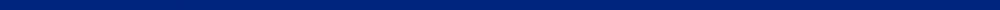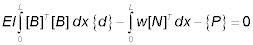CIVL 7117 - Homework AssignmentsAssignment Description Date Assigned 1 Do problems 2.4, 2.10, and 2.22 in your textbook August 30 2 Verify the global stiffness matrix for a three-dimensional bar. Hint: First, expand T* to a 6 x 6 square matrix, then expand 6 x 6 square matrix by adding the appropriate rows and columns of zeros, and finally, perform the matrix triple product k = TT September 5 3a Do problems 3.4, 3.10, 3.12, 3.15a,b, 3.18, 3.23, 3.37, 3.43, and 3.48 in your textbook September 10 3b Do problems 3.50, and 3.55 in your textbook September 12 4 Use SAP2000 and solve problems 3.63 and 3.67. September 12 5 Do problem B.9 in your textbook September 12 6 Solve the following truss problems: a 15-bar truss; a 25-bar truss; and a 72-bar truss.  You may use SAP2000 to do truss analysis. September 12 7 Verify the four beam element equations are contained in the following matrix expression.September 24 8 Do problems 4.10, 4.12, 4.18, 4.22, 4.40, and 4.47 in your textbook September 24 9 Work problem 4.36 in your textbook using the SAP2000. Attempt to select the lightest standard W section to support the loads for the beam. The bending stress must not exceed 160 MPa and the allowable deflection must not exceed (L = 6 m)/360 September 24 10 Do problems 5.1, 5.7, 5.15, 5.28, and 5.51 in your textbook. October 1 11 Do problems 5.20, 5.23, 5.25, 5.35, and 5.53 in your textbook. You may use the SAP2000 to do frame analysis. October 3 12 Do problems 6.6a, 6.6c, 6.7, 6.10a-c, 6.11, and 6.13. October 10 13 Rework the plane stress problem given on page 364 in your textbook using either FEM_2Dor3D_linelast_standard from Brower's MATLAB code to do analysis or Camp's CST code. Start with the simple two element model. Continuously refine your discretization by a factor of two each time until your FEM solution is in agreement with the exact solution for both displacements and stress. How many elements did you need? October 10 14 Do problems 7.2, 7.3, and 7.4 in your textbook. October 17 15 Work problems 7.13, 7.16, and 7.22 in your textbook. - Cancelled October 17 16 Work problems 8.3, 8.6, and 8.7 in your textbook. October 22 17 Rework the plane stress problem given on page 364 and 6.13 in your textbook using Camp's LST code. Start with the simple two element model. Continuously refine your discretization by a factor of two each time until your FEM solution is in agreement with the exact solution for both displacements and stress. How many elements did you need? October 22 18 Work problems 9.2, 9.3, and 9.5 in your textbook. October 31 19 Work 9.16 your textbook using Camp's axisymmetric CST code. October 31 20 Work problems 10.1, 10.6a, 10.8, 10.15dg, and 10.17b in your textbook. November 7 21 Write a computer program to evaluation of the [k] stiffness matrix for the Q4 element by Gaussian quadrature (click here for an Excel file containing some 1 to 7 Gauss points). Check your stiffness matrix values with the Example 10.4 in the textbook. In addition, develop your code in such a way that it could be easily extended to the Q8, Q9, and Q12 elements. November 7 22 Work problems 12.1 and 12.5 in your textbook using SAP2000. November 14 23 Work problems 16.6 and 16.11c in your textbook. November 28 24 Work problems 16.14 and 16.16 in your textbook using SAP2000. November 28 25 Work problems 11.1, 11.3, 11.6a, and 11.9 in your textbook. November 30 26 Use solid elements in SAP2000 to solve problem 11.14 in your textbook. November 30

This web site was originally developed by Charles Camp for CIVL 7117.
This site is Maintained by the Department of Civil Engineering at the University of Memphis.